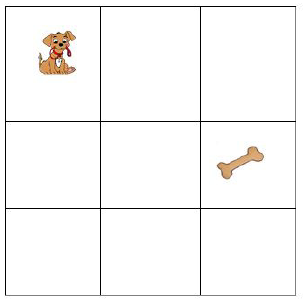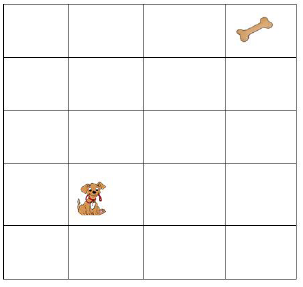Skip to Content
Use algorithms and related testing procedures to identify and correct errors - Maths Curriculum Companion - Department of Education & Training

## Teaching Context

### Victorian Curriculum

Use algorithms and related testing procedures to identify and correct errors (VCMNA282)

VCAA Sample Program: A set of sample programs covering the Victorian Curriculum Mathematics.

VCAA Mathematics glossary: A glossary compiled from subject-specific terminology found within the content descriptions of the Victorian Curriculum Mathematics.

##### Achievement standards

Students use efficient mental and written strategies to make estimates and carry out the four operations with integers, and apply the index laws to whole numbers. They identify and describe rational and irrational numbers in context.

Students estimate answers and solve everyday problems involving profit and loss rates, ratios and percentages, with and without the use of digital technology. They simplify a variety of algebraic expressions and connect expansion and factorisation of linear expressions.

Students solve linear equations and graph linear relationships on the Cartesian plane.

## Teaching ideas

### Developing divisibility tests

Tab Content

This activity introduces students to search and sort algorithms. They will develop algorithms to test the number for divisibility and apply the algorithm to search and sort numbers that can be divided.

Advise the class that they will be developing divisibility tests for numbers 2-10. Ask the class what a divisibility test is and whether they can identify some of them.

(Students may identify the simpler tests such as dividing by 10, 5 or 2).

Ask students to pair up. Their task is to determine divisibility tests for numbers 2-10. These tests (algorithms) will then be used to determine what a number is divisible by.

Note: students may become stuck at finding a divisibility test for 7. You may like to set it as a challenge for a pair to solve by the end of the lesson or allow students to research it on the internet.

The second part of their next task is to write a four-digit number (or larger) that applies to each of their 9 algorithms they have just developed. They should justify why each four-digit number selected meets the criteria for each divisibility test.

E.g. 4540 is divisible by 4 as the last two digits (40) are divisible by 4

7104 is divisible by 8 as the last three digits (104) are divisible by 8

Discuss with students:

• Which divisibility tests were more challenging to determine? Why?
• How did you go about testing your ideas for different divisibility tests?
• How did you know when you had a correct algorithm?

### Test it out: Problem-solving

Tab Content

This problem-solving task is intended once students have been introduced to divisibility tests and have determined algorithms to test divisibility.

Pose both questions to students. This could be used as a starter for a lesson or as a revision for divisibility tests.

• How many numbers can be divided into 2160? What are the numbers? Show justifications for each number selected using the algorithms for divisibility.
• What is the smallest number that can be divided by all the numbers from 1 to 10?

### Find the bug and rewrite the code

Tab Content

This activity is designed to show students where a code will fail and how they can modify the code to correct the error.

Ask students how they solve problems:

• How do you fix something that isn’t working?
• Do you follow a series of steps to try and find the problem?

Your task today is to fix some puzzles and codes that have already been done.

Each of the puzzles and codes has a ‘bug’ in it and it’s your job to debug them so they work properly.

Part 1: The dog wants to get to the bone. Fix the codes in both puzzles so the dog can reach the bone. The dog can move up, down, forward and backward.

Identify where the error/s are and use the minimum number of ‘coding lines’ required to fix the problem. You may use loops (repeat a step) if necessary.

PUZZLE 1• START
• MOVE FORWARD
• MOVE DOWN
• MOVE DOWN
• MOVE FORWARD

The error is in the third line. The possibilities to fix the code are:

START

MOVE FORWARD

MOVE DOWN

MOVE FORWARD

END

PUZZLE 2• START
• MOVE UP
• REPEAT TWICE
• MOVE FORWARD
• REPEAT ONCE
• MOVE DOWN
• END

The last line (move down) is incorrect. The possibilities to fix the code are:

START                      START

REPEAT 3 TIMES       REPEAT 2 TIMES

MOVE UP                  MOVE FORWARD

REPEAT 2 TIMES       REPEAT 3 TIMES

MOVE FORWARD       MOVE UP

END                         END

(REMEMBER TO USE THE MINIMUM NUMBER OF CODING LINES POSSIBLE)

Once students have attempted Part 1 have them pair up with another student and discuss their debugging attempts. Ask students to discuss the questions below.

• 'Did you write the same code?'
• 'If the codes are different do they both work? (Does the dog reach the bone?)'
• 'Are both codes written using the same amount of coding lines?'
• 'Can you find any other solutions to fix the errors that would use less coding lines?'

Part 2:

Working with their pair for this section advise students they will be debugging codes written in pseudo code. Their task is to calculate the current output, identify where the error is and how the code can be written to fix the error. They will then test their solutions to ensure the correct output is achieved.

CODE 1: This pseudo code is meant to find the difference between two numbers.

1. START

2. SET a = 14

3. SET b = 6

4. SET c = a + b

5. OUTPUT c

6. END

These questions may assist students who find it challenging to get started. You may choose to show these at the beginning or once students have attempted to debug the code on their own.

i) What is the output?

ii) What is the difference between 14 and 6?

iii) Which step contains the bug?

iv) Debug the code (rewrite the code without the error)

CODE 2: This pseudo code is meant to find the average of two numbers.

1. START

2. SET d = 10

3. SET e = 20

4. SET f = (d - e)/2

5. OUTPUT f

6. END

i) What is the output?

ii) What is the average of 10 and 20?

iii) Which step contains the bug?

iv) Debug the code (rewrite the code without the error)

To finish up the activity bring the class together for a final discussion.

• What do you learn today?
• How would you explain what you have learned to a friend?
• Did you use any helpful strategies to identify the error? What were they?

Alternatively, for a further task you may like to include Part 3. It could be completed in class or as a homework task.

Part 3: Your task is to write a code to solve an algorithm (E.g. adding two numbers). You will need to purposefully add in a bug to your code. Then you will swap your code with your partner and see if they can debug your work.

## Related content areas###### Previous Level
Design and implement mathematical algorithmsSet structures